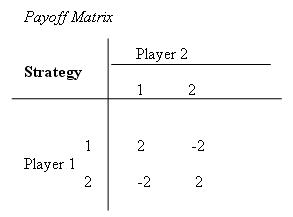# Two-Person Zero-Sum Games: Basic Concepts

Game theory provides a mathematical framework for analyzing the decision-making processes and strategies of adversaries (or players) in different types of competitive situations. The simplest type of competitive situations are two-person, zero-sum games. These games involve only two players; they are called zero-sum games because one player wins whatever the other player loses.

## Example: Odds and Evens

Consider the simple game called odds and evens. Suppose that player 1 takes evens and player 2 takes odds. Then, each player simultaneously shows either one finger or two fingers. If the number of fingers matches, then the result is even, and player 1 wins the bet ($2). If the number of fingers does not match, then the result is odd, and player 2 wins the bet ($2). Each player has two possible strategies: show one finger or show two fingers. The payoff matrix shown below represents the payoff to player 1.## Basic Concepts of Two-Person Zero-Sum Games

This game of odds and evens illustrates important concepts of simple games.

• A two-person game is characterized by the strategies of each player and the payoff matrix.
• The payoff matrix shows the gain (positive or negative) for player 1 that would result from each combination of strategies for the two players. Note that the matrix for player 2 is the negative of the matrix for player 1 in a zero-sum game.
• The entries in the payoff matrix can be in any units as long as they represent the utility (or value) to the player.
• There are two key assumptions about the behavior of the players. The first is that both players are rational. The second is that both players are greedy meaning that they choose their strategies in their own interest (to promote their own wealth).